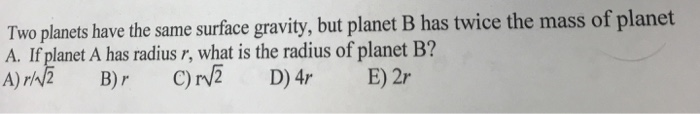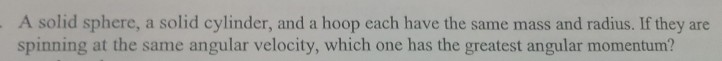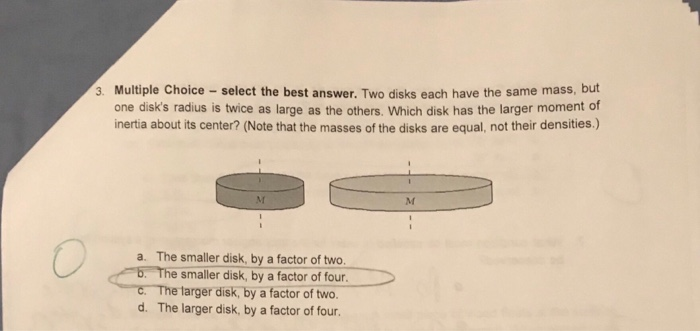# If Body A and Body B have the same mass, but Body A has a radius twice as large as Body B, which...

If Body A and Body B have the same mass, but Body A has a radius twice as large as Body B, which will have the higher escape velocity?
Body A
Body B
Neither

This Homework Help Question: "If Body A and Body B have the same mass, but Body A has a radius twice as large as Body B, which..." No answers yet.

We need 3 more requests to produce the answer to this homework help question. Share with your friends to get the answer faster!

0 /3 have requested the answer to this homework help question.

Once 3 people have made a request, the answer to this question will be available in 1-2 days.
All students who have requested the answer will be notified once they are available.
##### Add Answer of: If Body A and Body B have the same mass, but Body A has a radius twice as large as Body B, which...
Similar Homework Help Questions
• ### Astronomy HELP

If Body A and Body B have the same mass, but Body A has a radius twice as large as Body B, which will have the higher escape velocity?Body ABody BNeitherAbout the Same4. According to the lab, which of the following plays a secondary role to the retention of a planetary atmosphere?The mass of the solar system bodyThe mass of the gas molecules in the atmosphereThe temperature of the surface and atmosphereThe strength of the magnetic fieldThe radius of the...

• ### Two planets have the same surface gravity, but planet B has twice the mass of planet...Two planets have the same surface gravity, but planet B has twice the mass of planet A. If planet A has radius r, what is the radius of planet B? A) B)r C) We were unable to transcribe this image

• ### - A solid sphere, a solid cylinder, and a hoop each have the same mass and radius. If they are spinning at the same...- A solid sphere, a solid cylinder, and a hoop each have the same mass and radius. If they are spinning at the same angular velocity, which one has the greatest angular momentum?

• ### The specific brain mass is the brain mass divided by the body mass.Mammal A has twice...

The specific brain mass is the brain mass divided by the body mass.Mammal A has twice the body mass of mammal B. What is the ratio of mammal A's specific brain mass to mammal B's? Report your answer using two significant figures.

• ### The specific brain mass is the brain mass divided by the body mass.Mammal A has twice...

The specific brain mass is the brain mass divided by the body mass.Mammal A has twice the body mass of mammal B. What is the ratio of mammal A's specific brain mass to mammal B's? Report your answer using two significant figures.

• ### The specific brain mass is the brain mass divided by the body mass.Mammal A has twice...

The specific brain mass is the brain mass divided by the body mass.Mammal A has twice the body mass of mammal B. What is the ratio of mammal A's specific brain mass to mammal B's? Report your answer using two significant figures.

• ### The specific brain mass is the brain mass divided by the body mass.Mammal A has twice...

The specific brain mass is the brain mass divided by the body mass.Mammal A has twice the body mass of mammal B. What is the ratio of mammal A's specific brain mass to mammal B's? Report your answer using two significant figures.

• ### 3. Multiple Choice - select the best answer. Two disks each have the same mass, but...3. Multiple Choice - select the best answer. Two disks each have the same mass, but one disk's radius is twice as large as the others. Which disk has the larger moment of inertia about its center? (Note that the masses of the disks are equal, not their densities.) a. The smaller disk, by a factor of two smaller disk, by a factor of four. The targer disk, by a factor of two d. The larger disk, by a factor...

• ### A solid cylinder with a radius of 4.0 cm has the same mass as a solid...

A solid cylinder with a radius of 4.0 cm has the same mass as a solid sphere of radius R. If the cylinder and sphere have the same moment of inertia about their centers, what is the sphere's radius?

• ### help finding mass

suppose the escape velocity at the surface of the earth isapproximately 8 km/s.What is the mass, in units of Earth'smass, of a planet with twice the radius ofearth for which theescape speed is twice that of Earth?

Need Online Homework Help?